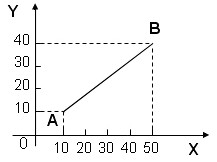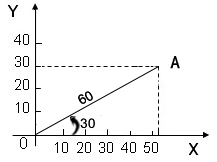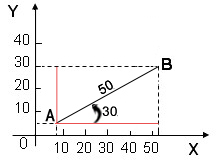How Can We Help?

Search for answers or browse our knowledge base.

## Drawing

You can accurately draw shapes by entering Absolute coordinates. coordinate, Relative coordinate, Absolute polar coordinate and Relative polar coordinate. The following example, to draw a straight line.

1. Absolute coordinate The coordinate values are calculated with relative to a fixed point origin O, expressed as: (X, Y).As figure: A(10,10) ,B(50,40),50 is the values of X axis coordinate, 40 is the values of Y axis coordinate. After entering the coordinates, need to press Enter key.

Example Demo:

Steps:

1.Click Menu【Draw > Line > Line】.

2.Pick the start point.

3.Enter the end point coordinates (150,100) by absolute coordinates , then press Enter key.

2. Relative coordinate: The coordinate values are calculated with relative to the previous point, expressed as: (rX, Y).As figure: point B is the point A of relative (incremental) coordinates, B(r40,30), 40 is the values of X axis coordinate, 30 is the values of Y axis coordinate.

Example Demo:

Steps:

1.Click Menu【Draw > Line > Line】.

2.Pick the start point.

3.Enter the end point coordinates (r150,100) by relative coordinate, then press Enter key.

3.Absolute polar coordinate: To fix apoint，that is relative to the coordinate origin O’s displacement distance, direction and angle, expressed as: (d<α).As figure: 60 is the value of distance from point A to origin O. 30 is the value of angle.

Example Demo:

Steps:

1.Click Menu【Draw > Line > Line】.

2.Pick the start point.

3.Enter the end point coordinates (100<30) by absolute polarcoordinate, then press Enter key.

4.Relative
polar coordinate
To fix aprevious point displacement distance, direction and angle, expressed as: (rd<α).As figure: 50 is the value of distance from point B to point A. 30 is the value of angle.

Example Demon:

Steps:

1.Click Menu【Draw > Line > Line】.

2.Pick the start point.

3.Enter the end point coordinates (r100<30) by relative
polar coordinate , then press Enter key.